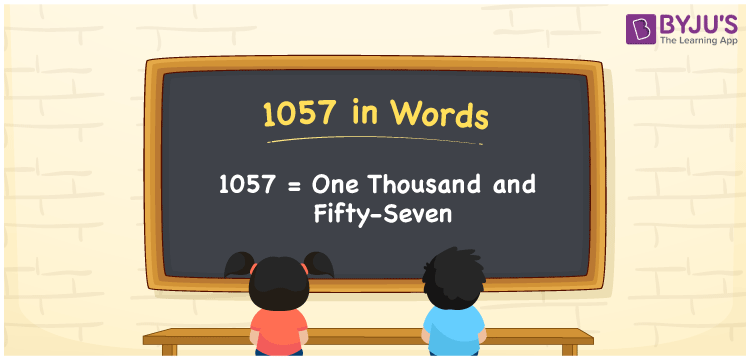# 1057 in Words

We can write 1057 in words as One Thousand and Fifty-seven. Suppose you bought a new specs that costs Rs. 1057, then you could say that “ I bought new specs for One Thousand and Fifty-seven rupees”. In this article, you will learn how to convert the number 1057 into words in an exciting way. The number 1057 is used in expressions that relate to money, days, distance, length, weight and so on.

 1057 in words One Thousand and Fifty-seven One Thousand and Fifty-seven in Numbers 1057

## 1057 in English Words

We generally write numbers in words using the English alphabet. Thus, we can read 1057 in English as “One Thousand and Fifty-seven”.## How to Write 1057 in Words?

In this section, you will learn how to write the number 1057 in words. The given number 1057 has four digits, so we should make a place value chart that represents the place value for all these four digits. The order of place values of digits in a number is in accordance with the Indian numbering system, such as:

• Ones
• Tens
• Hundreds
• Thousands
• Ten-thousands
• Hundred-thousands or Lakhs

This can be done as follows.

 Thousands Hundreds Tens Ones 1 0 5 7

Thus, we can write the expanded form as:

1 × Thousand + 0 × Hundred + 5 × Ten + 7 × One

= 1 × 1000 + 0 × 100 + 5 × 10 + 7 × 1

= 1057

= One thousand five hundred

1057 is a natural number that precedes 1058 and succeeds 1056.

1057 in words – One Thousand and Fifty-seven

Is 1057 an odd number? – Yes

Is 1057 an even number? – No

Is 1057 a perfect square number? – No

Is 1057 a perfect cube number? – No

Is 1057 a prime number? – No

Is 1057 a composite number? – Yes

## Frequently Asked Questions on 1057 in Words

Q1

### How do you write 1057 in English?

We can write 1057 in English words as “One Thousand and Fifty-seven”.
Q2

### How do you write 1057 in words for a cheque?

For a cheque, we generally write 1057 in words as One Thousand and Fifty-seven rupees only.
Q3

### What number is 1057 in words?

The number 1057 is One Thousand and Fifty-seven in words.